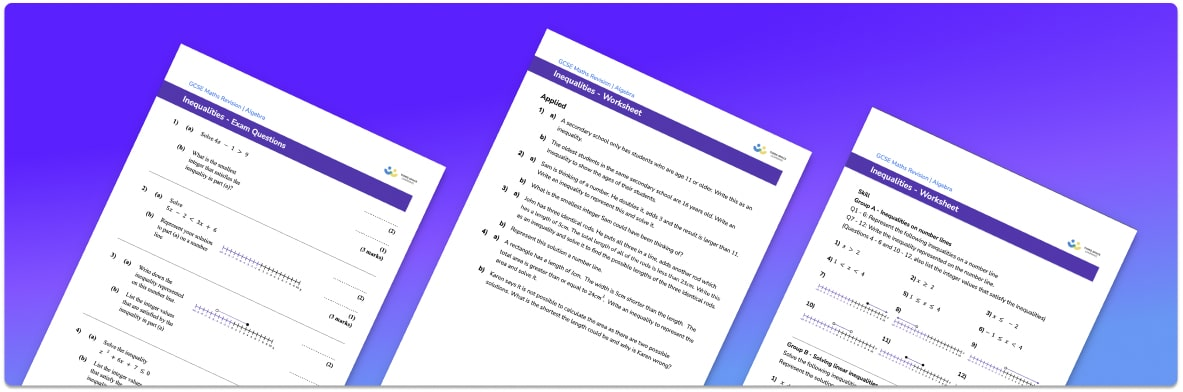# Inequalities Worksheet• Section 1 of the inequalities worksheet contains 20+ skills-based inequalities questions, in 3 groups to support differentiation
• Section 2 contains 3 applied inequalities questions with a mix of worded problems and deeper problem solving questions
• Section 3 contains 3 foundation and higher level GCSE exam style inequalities questions
• Answers and a mark scheme for all inequalities questions are provided
• Questions follow variation theory with plenty of opportunities for students to work independently at their own level
• All questions created by fully qualified expert secondary maths teachers
• Suitable for GCSE maths revision for AQA, OCR and Edexcel exam boards

• This field is for validation purposes and should be left unchanged.

You can unsubscribe at any time (each email we send will contain an easy way to unsubscribe). To find out more about how we use your data, see our privacy policy.

### Inequalities at a glance

Inequalities demonstrate relationships between two expressions that are not equal to each other. There are four types of inequalities: greater than >, less than <, greater than or equal to ≥ and less than or equal to ≤. For example, x>4 means x is greater than 4 , so x could be 5, 6, 7, … etc.

Single variable inequalities can be represented on a number line and we can also list the integer (whole number) solutions to inequalities.

Solving algebraic inequalities such as linear inequalities and quadratic inequalities is very similar to solving algebraic equations, but instead of using an equals sign we use an inequality symbol. As with linear equations, we can have one step inequalities, two step inequalities and unknowns on each side of the inequality. The solution to an inequality represents a range of values rather than one number.

Quadratic inequalities are initially dealt with in the same way as quadratic equations by calculating the roots of the equation by factorising or using the quadratic formula. These roots can then be plotted and the general graph can be sketched. The possible values for x can then be worked out by looking for the values of x where the graph is either above or below the x axis.

Looking forward, students can progress with more inequalities worksheets to additional algebra worksheets, for example a simplifying expressions worksheet or straight line graph worksheet.For more teaching and learning support on Algebra our GCSE maths lessons provide step by step support for all GCSE maths concepts.

## Do you have KS4 students who need more focused attention to succeed at GCSE?There will be students in your class who require individual attention to help them succeed in their maths GCSEs. In a class of 30, it’s not always easy to provide.

Help your students feel confident with exam-style questions and the strategies they’ll need to answer them correctly with our dedicated GCSE maths revision programme.

Lessons are selected to provide support where each student needs it most, and specially-trained GCSE maths tutors adapt the pitch and pace of each lesson. This ensures a personalised revision programme that raises grades and boosts confidence.

Find out more